# Simple Volatility EMV Strategy

Author: , Created: 2020-07-01 10:39:17, Updated:

# Summary

Unlike other technical indicators, “Ease of Movement Value” reflects changes in price, volume, and popularity. It is a technology that combines prices and volume changes. It measures the price change of unit volume, Forming a price volatility indicator. When the market gathers popularity and the transaction is active, it prompts a buying signal; when the trading volume is low, and the market energy is about to run out, it prompts a selling signal.

Simple volatility EMV is designed according to the principle of equal volume chart and compressed chart. Its core concept is: market price will consume a lot of energy only when the trend turns or is about to turn, and the external performance is that the trading volume becomes larger. When the price is rising, it will not consume too much energy due to the boosting effect. Although this idea is contrary to the view that both quantity and price rise, it does have its own unique features.

# EMV calculation formula

Step 1: Calculate mov_mid

Among them, TH represents the highest price of the day, TL represents the lowest price of the day, YH represents the highest price of the previous day, and YL represents the lowest price of the previous day. Then if MID> 0 means today’s average price is higher than yesterday’s average price.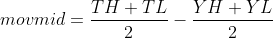Step 2: Calculate ratio

Among them, TVOL represents the trading volume of the day, TH represents the highest price of the day, and TL represents the lowest price of the day.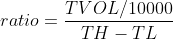Step 3: Calculate emv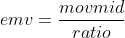# EMV usage

The author of EMV believes that the huge rise is accompanied by the rapid depletion of energy, and the rise often does not last too long; on the contrary, the moderate volume, which can save a certain amount of energy, often makes the rise last longer. Once an upward trend is formed, less trading volume can push prices up, and the value of EMV will increase. Once the downtrend market is formed, it is often accompanied by an infinite or small decline, and the value of EMV will decline. If the price is in a volatile market or the price rises and falls are accompanied by a large volume, the value of EMV will also be close to zero. So you will find that EMV is below the zero axis in most of the market, which is also a major feature of this indicator. From another perspective, EMV values ​​mega-trends and can generate sufficient profit.

The usage of EMV is quite simple, just look at whether EMV crosses the zero axis. When EMV is below 0, it represents a weak market; when EMV is above 0, it represents a strong market. When EMV changes from negative to positive, it should be bought; when EMV changes from positive to negative, it should be sold. Its characteristic is that it can not only avoid the shock market in the market, but also enter the market in time when the trend market starts. However, because EMV reflects the change in volume when prices change, it only has an effect on mid to long-term trends. For short-term or relatively short trading cycles, EMV’s effect is very poor.

# Strategy realization

Step 1: Write a strategy framework

``````# Strategy main function
def onTick():
pass

# Program entry
def main():
while True: # Enter infinite loop mode
onTick() # execution strategy main function
Sleep(1000) # sleep for 1 second
``````

FMZ.COM adopts the rotation training mode. First, you need to define a `main` function and an `onTick` function. The `main` function is the entry function of the strategy, and the program will execute the code line by line from the `main` function. In the `main` function, write a `while` loop and repeatedly execute the `onTick` function. All the core code of the strategy is written in the `onTick` function.

Step 2: Get position data

``````def get_position():
position = 0 # The number of assigned positions is 0
position_arr = _C(exchange.GetPosition) # Get array of positions
if len(position_arr)> 0: # If the position array length is greater than 0
for i in position_arr: # Traverse the array of positions
if i['ContractType'] =='IH000': # If the position symbol is equal to the subscription symbol
if i['Type']% 2 == 0: # if it is long position
position = i['Amount'] # Assign a positive number of positions
else:
position = -i['Amount'] # Assign the number of positions to be negative
return position # return position quantity
``````

Because in this strategy, only the number of real-time positions is used, in order to facilitate maintenance, `get_position` is used here to encapsulate the amount of positions. If the current position is long, it returns a positive number, and if the current position is short, it returns a negative number.

Step 3: Get K-line data

``````exchange.SetContractType('IH000') # Subscribe to futures variety
bars_arr = exchange.GetRecords() # Get K-line array
if len(bars_arr) <10: # If the number of K lines is less than 10
return
``````

Before obtaining specific K-line data, you must first subscribe to a specific trading contract, use the `SetContractType` function from FMZ.COM, and pass in the contract code. If you want to know other information about the contract, you can also use a variable to receive this data. Then use `GetRecords` function to get K-line data, because the returned is an array, so we use the variable `bars_arr` to accept it.

Step 4: Calculate emv

``````bar1 = bars_arr[-2] # Get the previous K-line data
bar2 = bars_arr[-3] # get the previous K-line data
# Calculate the value of mov_mid
mov_mid = (bar1['High'] + bar1['Low']) / 2-(bar2['High'] + bar2['Low']) / 2
if bar1['High'] != bar1['Low']: # If the dividend is not 0
# Calculate the value of ratio
ratio = (bar1['Volume'] / 10000) / (bar1['High']-bar1['Low'])
else:
ratio = 0
# If the value of ratio is greater than 0
if ratio> 0:
emv = mov_mid / ratio
else:
emv = 0
``````

Here, we do not use the latest price to calculate the value of EMV, but use the relatively lagging current K line to output the signal and place a K line to issue an order. The purpose of this is to make the backtest closer to real trading. We know that although the quantitative trading software is now very advanced, it is still difficult to completely simulate the real price tick environment, especially when faced with backtesting Bar-level long data, so this compromise method is used.

Step 5: Placing the orders

``````current_price = bars_arr[-1]['Close'] # latest price
position = get_position() # Get the latest position
if position> 0: # If you are holding long positions
if emv <0: # If the current price is less than teeth
exchange.Sell(round(current_price-0.2, 2), 1) # close long position
if position <0: # If you are holding short positions
if emv> 0: # If the current price is greater than the teeth
exchange.SetDirection("closesell") # Set the trading direction and type
exchange.Buy(round(current_price + 0.2, 2), 1) # close short position
if position == 0: # If there is no holding position
if emv> 0: # If the current price is greater than the upper lip
exchange.Buy(round(current_price + 0.2, 2), 1) # open long position
if emv <0: # if the current price is smaller than the chin
exchange.SetDirection("sell") # Set the trading direction and type
exchange.Sell(round(current_price-0.2, 2), 1) # open short position
``````

Before placing the order, we need to determine two data, one is the price of the order and the other is the current position status. The price of placing an order is very simple, just use the current closing price to add or subtract the minimum change price of the variety. Since we have used the `get_position` function to encapsulate the position, we can call it directly here. Finally, the position is opened and closed according to the positional relationship between the EMV and the zero axis.

# Strategy backtest

Backtest configuration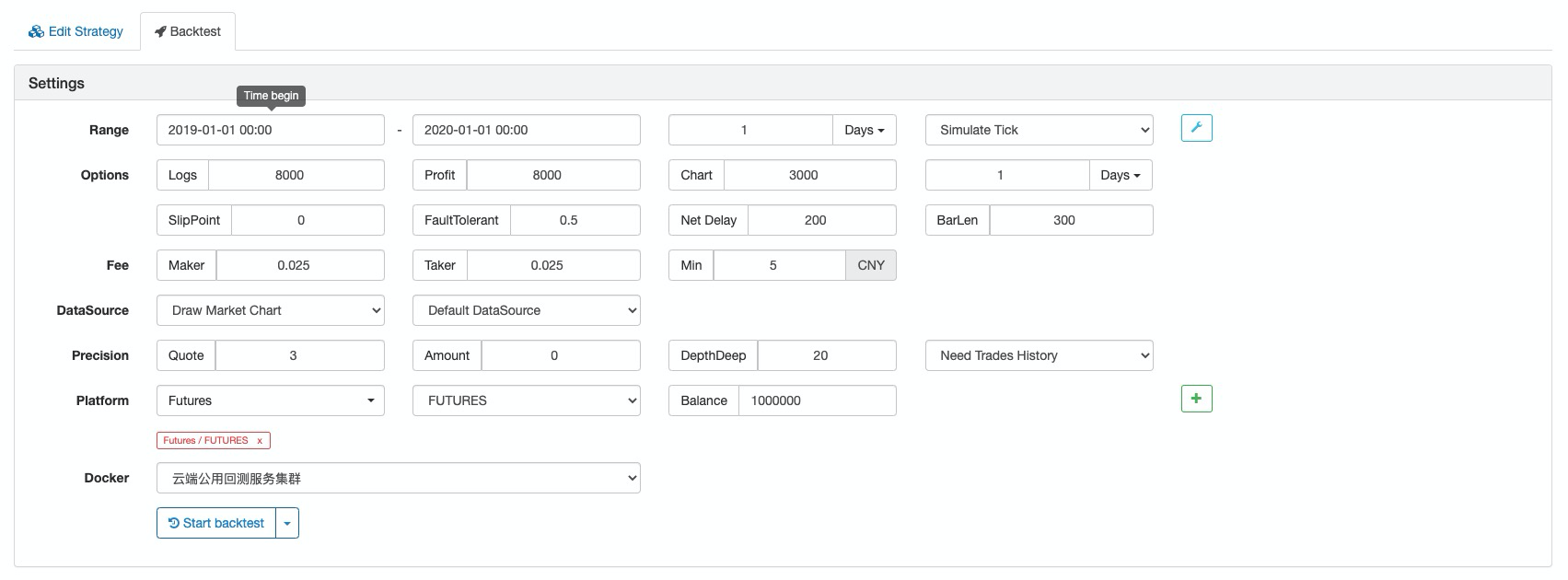Backtest log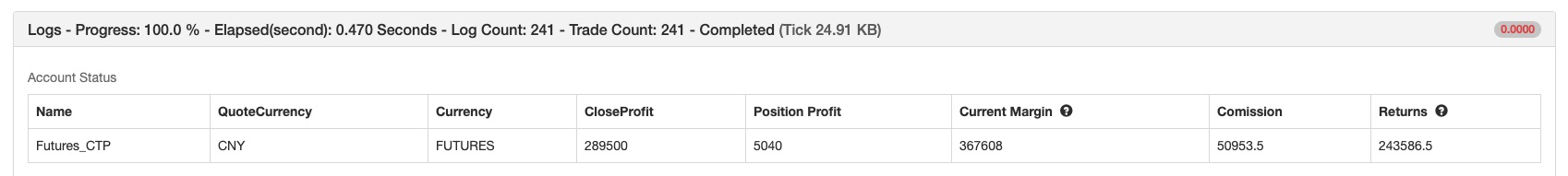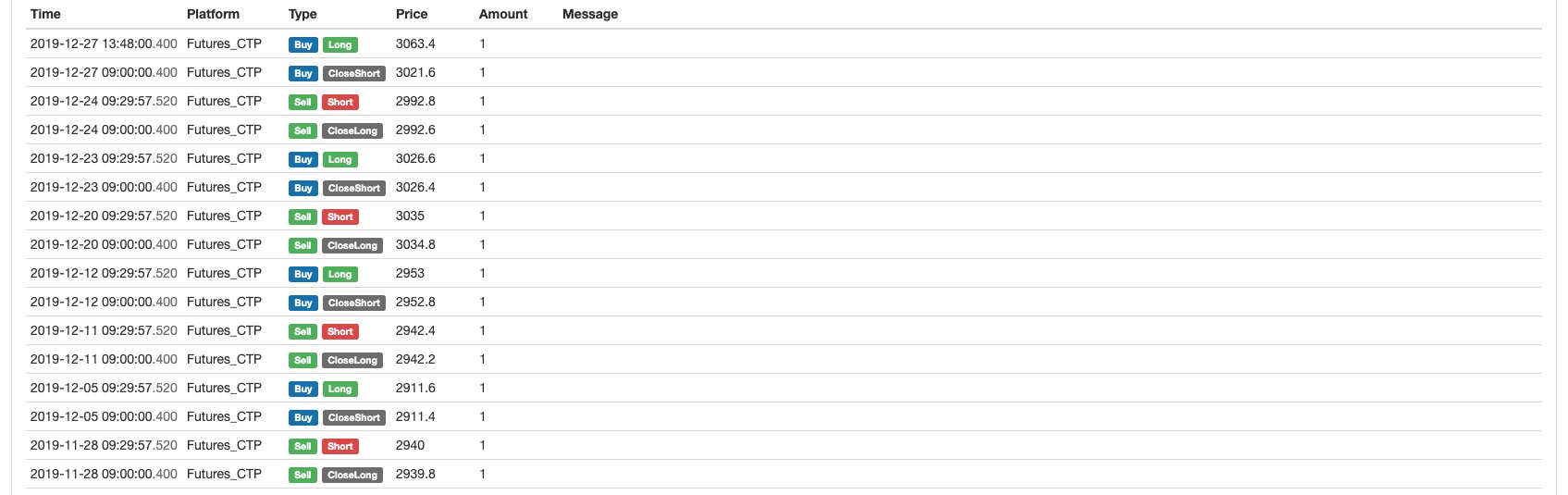Capital curve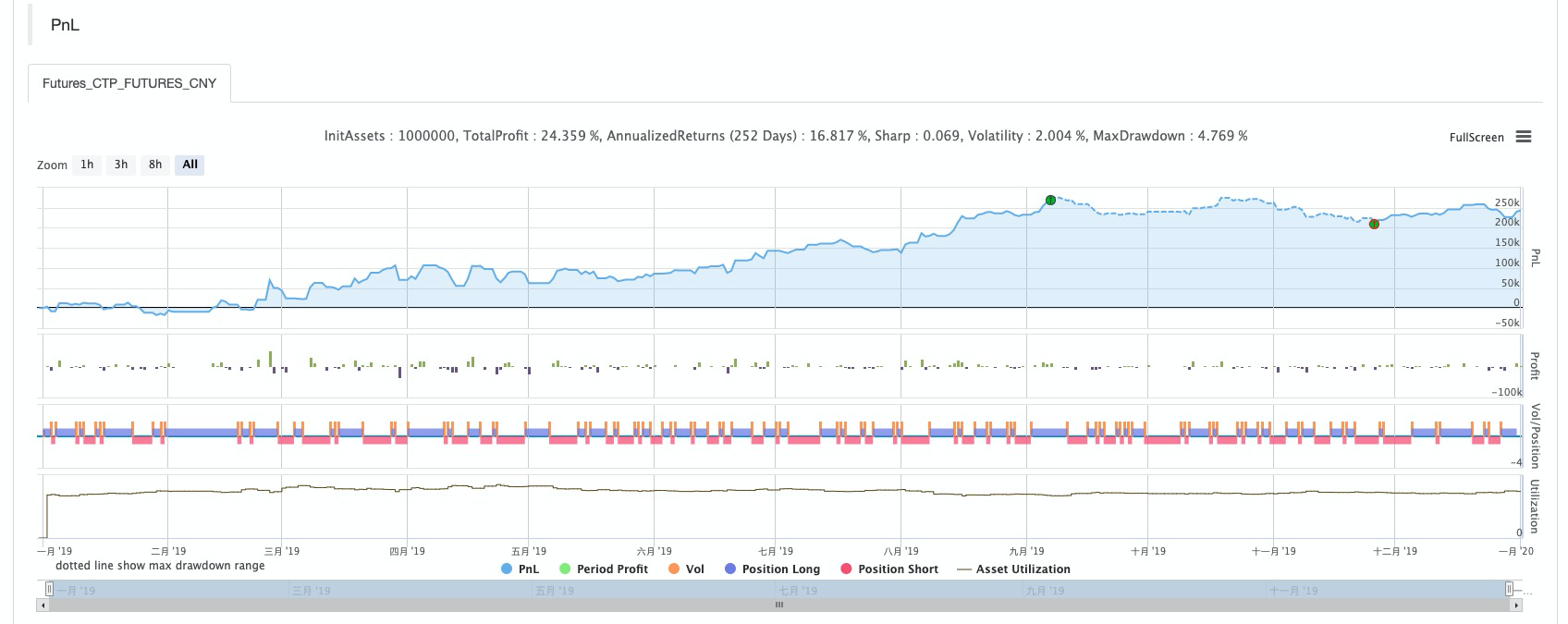# Complete strategy

``````# Backtest configuration
'''backtest
start: 2019-01-01 00:00:00
end: 2020-01-01 00:00:00
period: 1d
basePeriod: 1d
exchanges: [{"eid":"Futures_CTP","currency":"FUTURES"}]
'''

def get_position():
position = 0 # The number of assigned positions is 0
position_arr = _C(exchange.GetPosition) # Get array of positions
if len(position_arr)> 0: # If the position array length is greater than 0
for i in position_arr: # Traverse the array of positions
if i['ContractType'] =='IH000': # If the position symbol is equal to the subscription symbol
if i['Type']% 2 == 0: # if it is long position
position = i['Amount'] # Assign a positive number of positions
else:
position = -i['Amount'] # Assign the number of positions to be negative
return position # return position quantity

# Strategy main function
def onTick():
# retrieve data
exchange.SetContractType('IH000') # Subscribe to futures
bars_arr = exchange.GetRecords() # Get K-line array
if len(bars_arr) <10: # If the number of K lines is less than 10
return

# Calculate emv
bar1 = bars_arr[-2] # Get the previous K-line data
bar2 = bars_arr[-3] # get the previous K-line data
# Calculate the value of mov_mid
mov_mid = (bar1['High'] + bar1['Low']) / 2-(bar2['High'] + bar2['Low']) / 2
if bar1['High'] != bar1['Low']: # If the dividend is not 0
# Calculate the value of ratio
ratio = (bar1['Volume'] / 10000) / (bar1['High']-bar1['Low'])
else:
ratio = 0
# If the value of ratio is greater than 0
if ratio> 0:
emv = mov_mid / ratio
else:
emv = 0

# Placing orders
current_price = bars_arr[-1]['Close'] # latest price
position = get_position() # Get the latest position
if position> 0: # If you are holding long positions
if emv <0: # If the current price is less than teeth
exchange.Sell(round(current_price-0.2, 2), 1) # close long position
if position <0: # If you are holding short positions
if emv> 0: # If the current price is greater than the teeth
exchange.SetDirection("closesell") # Set the trading direction and type
exchange.Buy(round(current_price + 0.2, 2), 1) # close short position
if position == 0: # If there is no holding position
if emv> 0: # If the current price is greater than the upper lip
exchange.Buy(round(current_price + 0.2, 2), 1) # open long position
if emv <0: # if the current price is smaller than the chin
exchange.SetDirection("sell") # Set the trading direction and type
exchange.Sell(round(current_price-0.2, 2), 1) # open short position

# Program entry
def main():
while True: # Enter infinite loop mode
onTick() # execution strategy main function
Sleep(1000) # sleep for 1 second
``````

The complete strategy has been published to the strategy square of the FMZ.COM website, and it can be used by clicking Copy. https://www.fmz.com/strategy/213636

# To sum up

Through this course of study, we can see that EMV is contrary to ordinary traders, but it is not unreasonable. Because EMV introduces volume data, it is more effective than other technical indicators that use price calculations to find out what’s behind the price. Each strategy has different characteristics. Only by fully understanding the advantages and disadvantages of different strategies and removing the dross and extracting its essence can we go further from success.

More